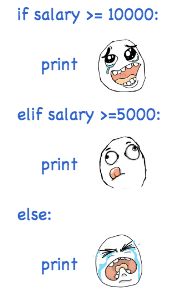# 条件判断和循环

### 条件判断

``````age = 20
if age >= 18:
``````

``````age = 3
if age >= 18:
else:
print 'teenager'
``````

``````age = 3
if age >= 18:
elif age >= 6:
print 'teenager'
else:
print 'kid'
``````

`elif``else if`的缩写，完全可以有多个`elif`，所以`if`语句的完整形式就是：

``````if <条件判断1>:
<执行1>
elif <条件判断2>:
<执行2>
elif <条件判断3>:
<执行3>
else:
<执行4>
``````

`if`语句执行有个特点，它是从上往下判断，如果在某个判断上是`True`，把该判断对应的语句执行后，就忽略掉剩下的`elif``else`，所以，请测试并解释为什么下面的程序打印的是`teenager`

``````age = 20
if age >= 6:
print 'teenager'
elif age >= 18:
else:
print 'kid'
``````

`if`判断条件还可以简写，比如写：

``````if x:
print 'True'
``````

### 循环

Python的循环有两种，一种是for...in循环，依次把list或tuple中的每个元素迭代出来，看例子：

``````names = ['Michael', 'Bob', 'Tracy']
for name in names:
print name
``````

``````Michael
Bob
Tracy
``````

``````sum = 0
for x in [1, 2, 3, 4, 5, 6, 7, 8, 9, 10]:
sum = sum + x
print sum
``````

``````>>> range(5)
[0, 1, 2, 3, 4]
``````

range(101)就可以生成0-100的整数序列，计算如下：

``````sum = 0
for x in range(101):
sum = sum + x
print sum
``````

``````sum = 0
n = 99
while n > 0:
sum = sum + n
n = n - 2
print sum
``````

### 再议raw_input

``````birth = raw_input('birth: ')
if birth < 2000:
print '00前'
else:
print '00后'
``````

``````>>> birth
'1982'
>>> '1982' < 2000
False
>>> 1982 < 2000
True
``````

``````birth = int(raw_input('birth: '))
``````

``````Traceback (most recent call last):
...
ValueError: invalid literal for int() with base 10: 'abc'
``````

### 小结